Скачать презентацию NP-Completeness Poly time algorithm input size n

fcb4c8d16ab501159531b3927e781118.ppt

• Количество слайдов: 58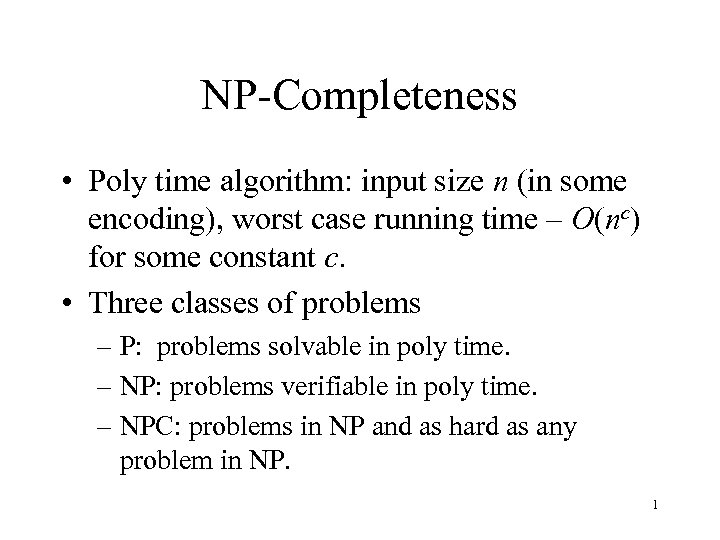NP-Completeness • Poly time algorithm: input size n (in some encoding), worst case running time – O(nc) for some constant c. • Three classes of problems – P: problems solvable in poly time. – NP: problems verifiable in poly time. – NPC: problems in NP and as hard as any problem in NP. 1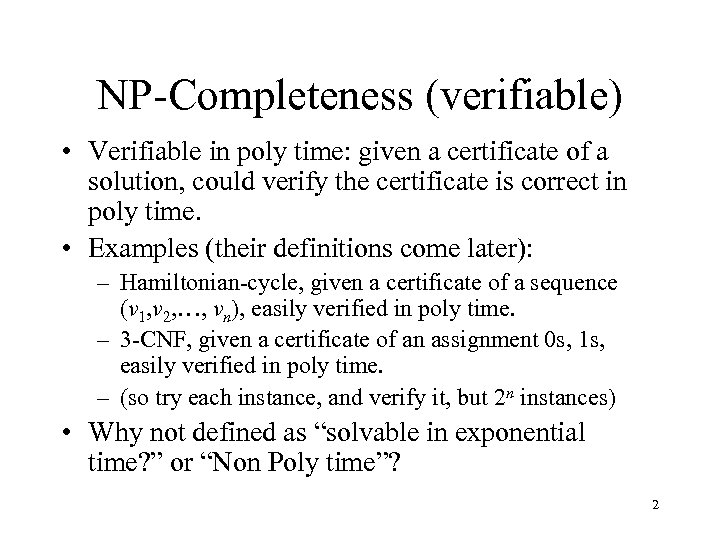NP-Completeness (verifiable) • Verifiable in poly time: given a certificate of a solution, could verify the certificate is correct in poly time. • Examples (their definitions come later): – Hamiltonian-cycle, given a certificate of a sequence (v 1, v 2, …, vn), easily verified in poly time. – 3 -CNF, given a certificate of an assignment 0 s, 1 s, easily verified in poly time. – (so try each instance, and verify it, but 2 n instances) • Why not defined as “solvable in exponential time? ” or “Non Poly time”? 2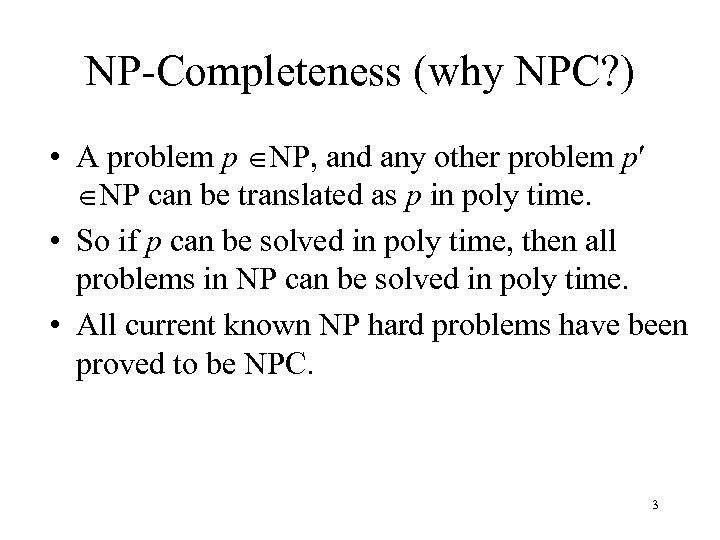NP-Completeness (why NPC? ) • A problem p NP, and any other problem p NP can be translated as p in poly time. • So if p can be solved in poly time, then all problems in NP can be solved in poly time. • All current known NP hard problems have been proved to be NPC. 3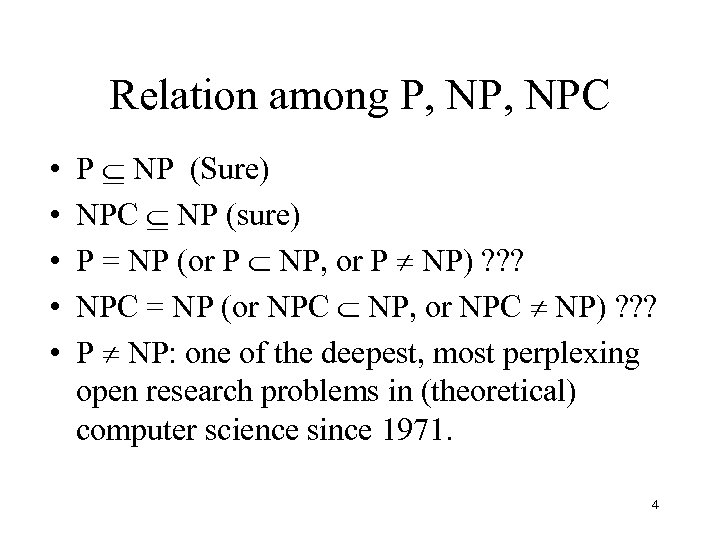Relation among P, NPC • • • P NP (Sure) NPC NP (sure) P = NP (or P NP, or P NP) ? ? ? NPC = NP (or NPC NP, or NPC NP) ? ? ? P NP: one of the deepest, most perplexing open research problems in (theoretical) computer science since 1971. 4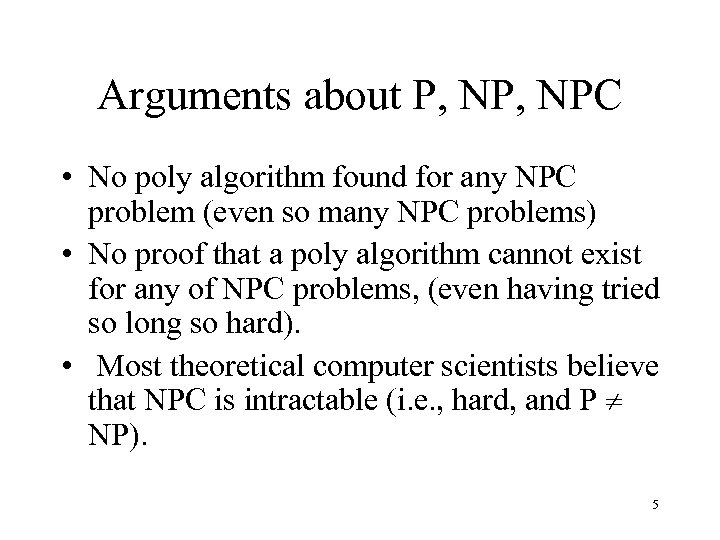Arguments about P, NPC • No poly algorithm found for any NPC problem (even so many NPC problems) • No proof that a poly algorithm cannot exist for any of NPC problems, (even having tried so long so hard). • Most theoretical computer scientists believe that NPC is intractable (i. e. , hard, and P NP). 5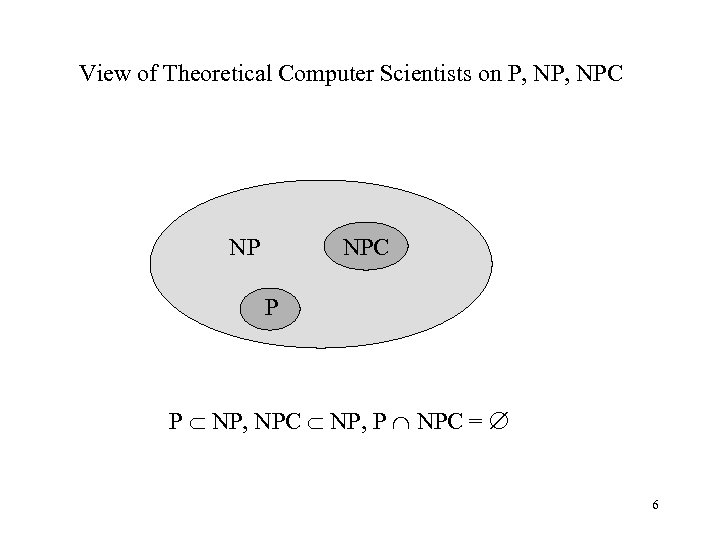View of Theoretical Computer Scientists on P, NPC NP P P NP, NPC NP, P NPC = 6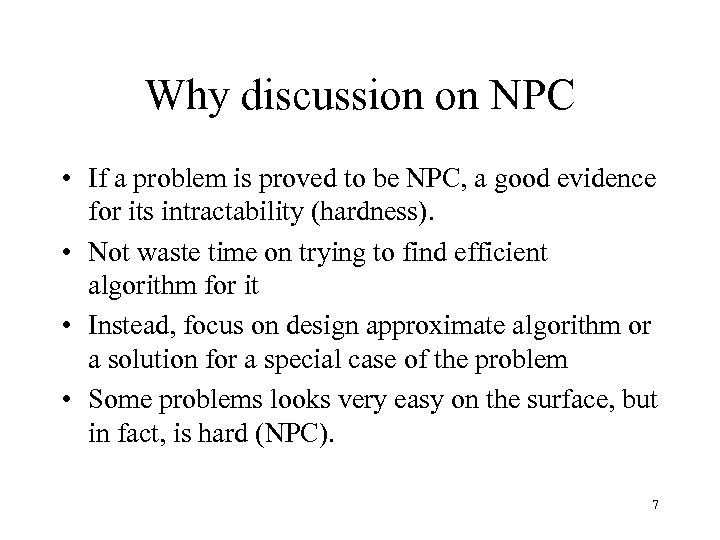Why discussion on NPC • If a problem is proved to be NPC, a good evidence for its intractability (hardness). • Not waste time on trying to find efficient algorithm for it • Instead, focus on design approximate algorithm or a solution for a special case of the problem • Some problems looks very easy on the surface, but in fact, is hard (NPC). 7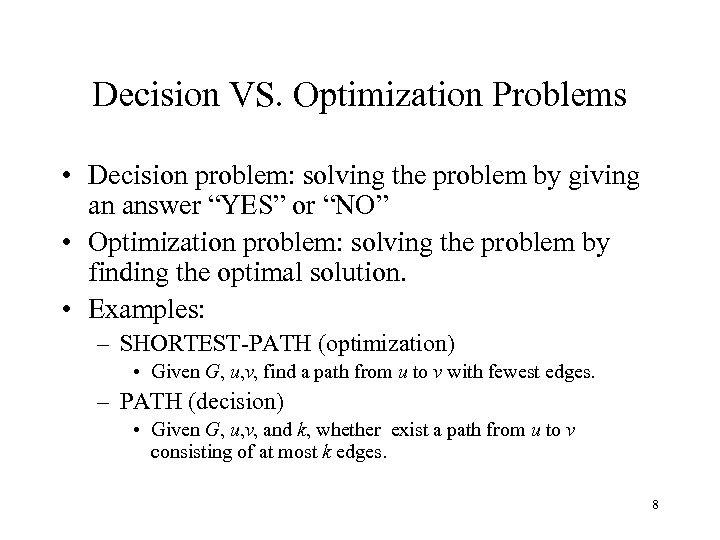Decision VS. Optimization Problems • Decision problem: solving the problem by giving an answer “YES” or “NO” • Optimization problem: solving the problem by finding the optimal solution. • Examples: – SHORTEST-PATH (optimization) • Given G, u, v, find a path from u to v with fewest edges. – PATH (decision) • Given G, u, v, and k, whether exist a path from u to v consisting of at most k edges. 8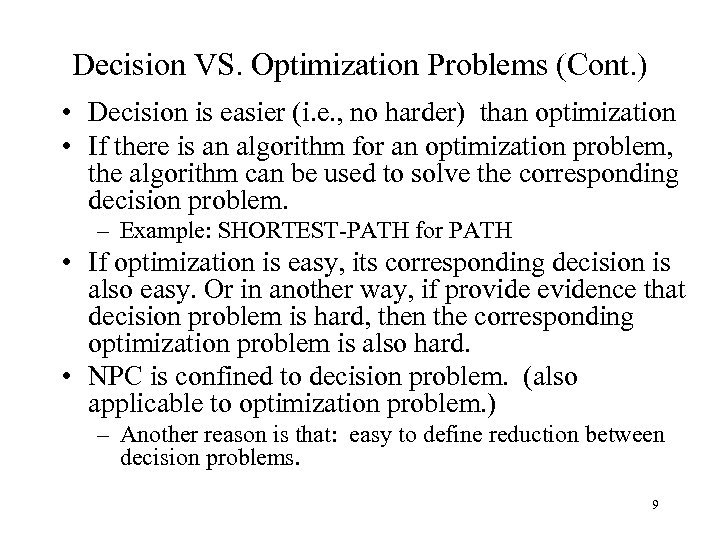Decision VS. Optimization Problems (Cont. ) • Decision is easier (i. e. , no harder) than optimization • If there is an algorithm for an optimization problem, the algorithm can be used to solve the corresponding decision problem. – Example: SHORTEST-PATH for PATH • If optimization is easy, its corresponding decision is also easy. Or in another way, if provide evidence that decision problem is hard, then the corresponding optimization problem is also hard. • NPC is confined to decision problem. (also applicable to optimization problem. ) – Another reason is that: easy to define reduction between decision problems. 9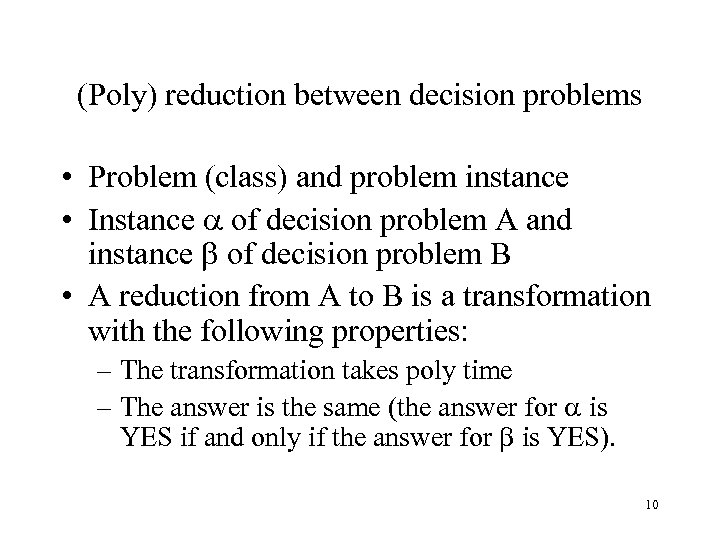(Poly) reduction between decision problems • Problem (class) and problem instance • Instance of decision problem A and instance of decision problem B • A reduction from A to B is a transformation with the following properties: – The transformation takes poly time – The answer is the same (the answer for is YES if and only if the answer for is YES). 10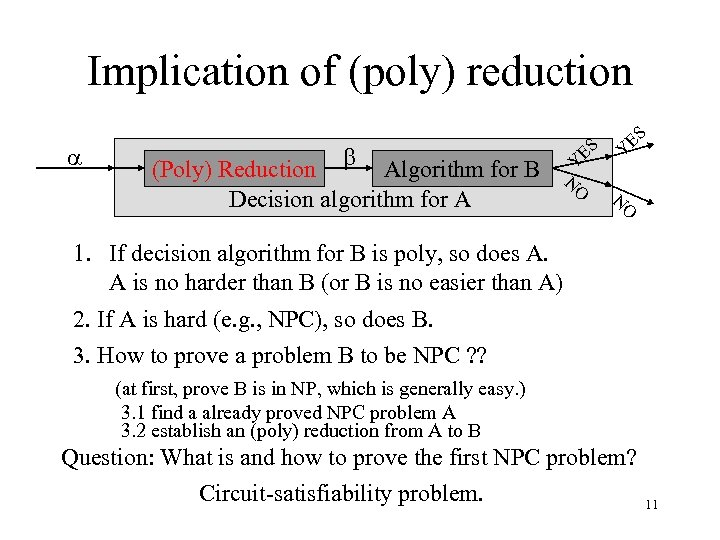ES Y O N (Poly) Reduction Algorithm for B Decision algorithm for A Y ES Implication of (poly) reduction 1. If decision algorithm for B is poly, so does A. A is no harder than B (or B is no easier than A) 2. If A is hard (e. g. , NPC), so does B. 3. How to prove a problem B to be NPC ? ? (at first, prove B is in NP, which is generally easy. ) 3. 1 find a already proved NPC problem A 3. 2 establish an (poly) reduction from A to B Question: What is and how to prove the first NPC problem? Circuit-satisfiability problem. 11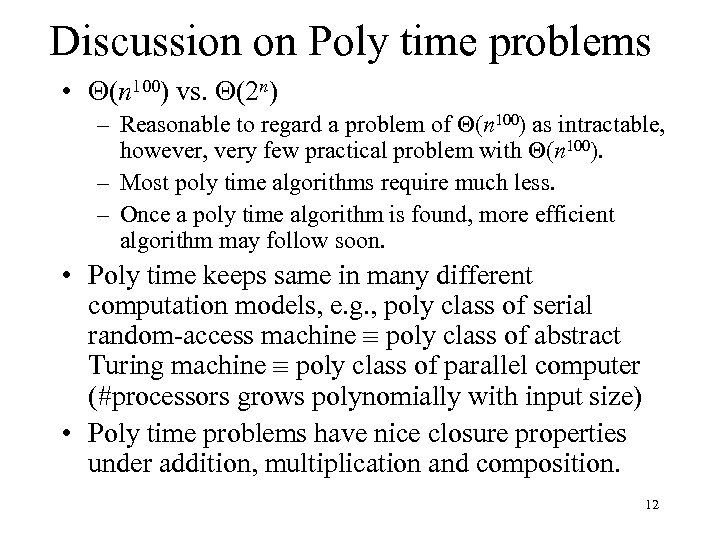Discussion on Poly time problems • (n 100) vs. (2 n) – Reasonable to regard a problem of (n 100) as intractable, however, very few practical problem with (n 100). – Most poly time algorithms require much less. – Once a poly time algorithm is found, more efficient algorithm may follow soon. • Poly time keeps same in many different computation models, e. g. , poly class of serial random-access machine poly class of abstract Turing machine poly class of parallel computer (#processors grows polynomially with input size) • Poly time problems have nice closure properties under addition, multiplication and composition. 12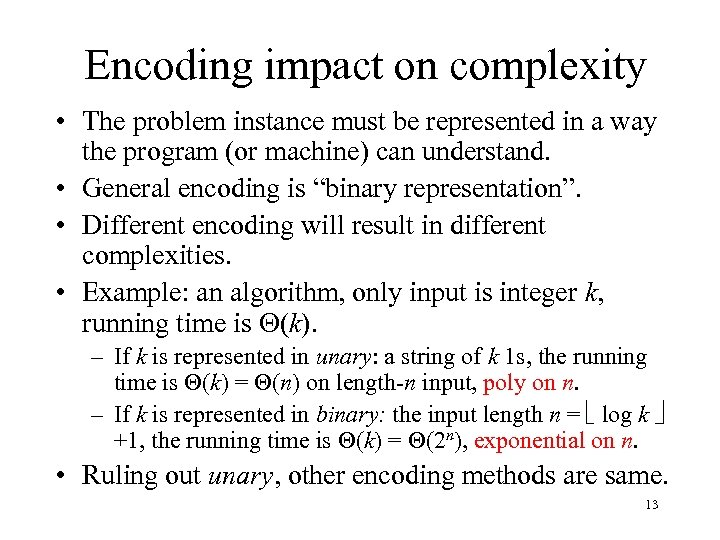Encoding impact on complexity • The problem instance must be represented in a way the program (or machine) can understand. • General encoding is “binary representation”. • Different encoding will result in different complexities. • Example: an algorithm, only input is integer k, running time is (k). – If k is represented in unary: a string of k 1 s, the running time is (k) = (n) on length-n input, poly on n. – If k is represented in binary: the input length n = log k +1, the running time is (k) = (2 n), exponential on n. • Ruling out unary, other encoding methods are same. 13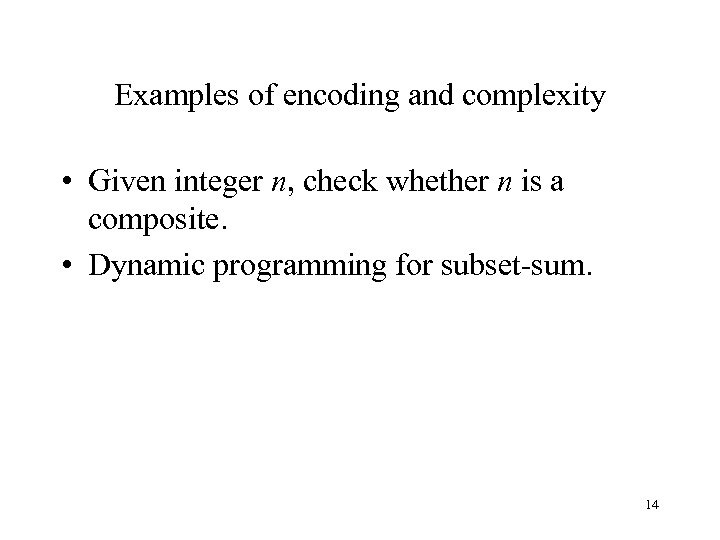Examples of encoding and complexity • Given integer n, check whether n is a composite. • Dynamic programming for subset-sum. 14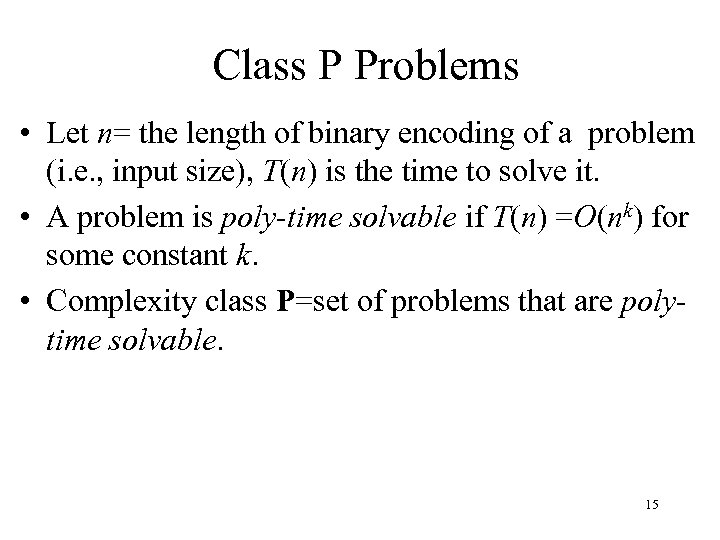Class P Problems • Let n= the length of binary encoding of a problem (i. e. , input size), T(n) is the time to solve it. • A problem is poly-time solvable if T(n) =O(nk) for some constant k. • Complexity class P=set of problems that are polytime solvable. 15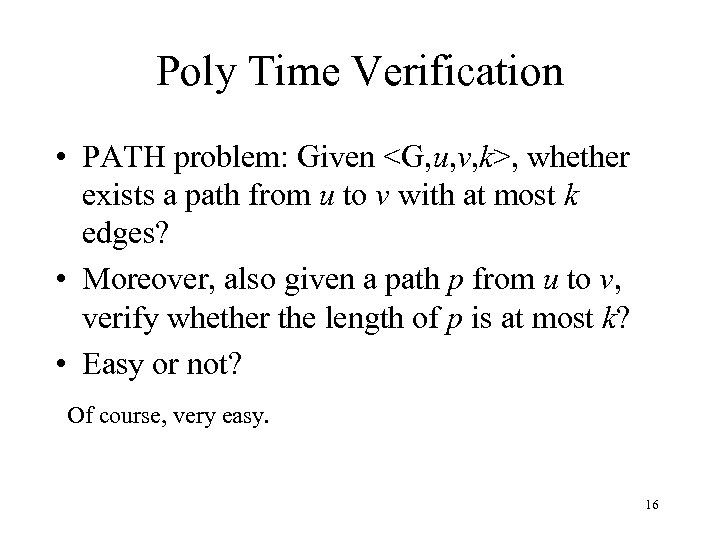Poly Time Verification • PATH problem: Given , whether exists a path from u to v with at most k edges? • Moreover, also given a path p from u to v, verify whether the length of p is at most k? • Easy or not? Of course, very easy. 16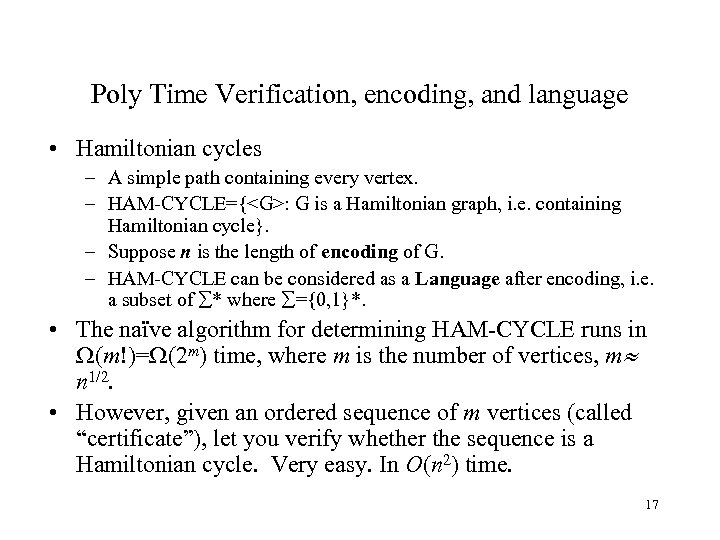Poly Time Verification, encoding, and language • Hamiltonian cycles – A simple path containing every vertex. – HAM-CYCLE={: G is a Hamiltonian graph, i. e. containing Hamiltonian cycle}. – Suppose n is the length of encoding of G. – HAM-CYCLE can be considered as a Language after encoding, i. e. a subset of * where ={0, 1}*. • The naïve algorithm for determining HAM-CYCLE runs in (m!)= (2 m) time, where m is the number of vertices, m n 1/2. • However, given an ordered sequence of m vertices (called “certificate”), let you verify whether the sequence is a Hamiltonian cycle. Very easy. In O(n 2) time. 17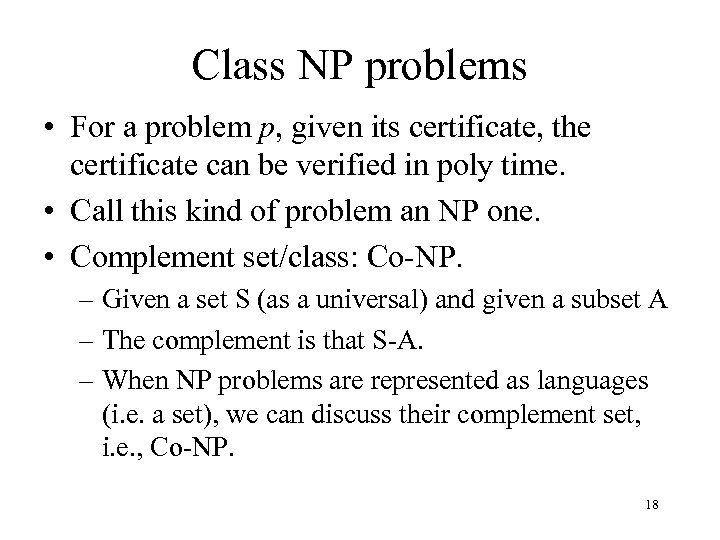Class NP problems • For a problem p, given its certificate, the certificate can be verified in poly time. • Call this kind of problem an NP one. • Complement set/class: Co-NP. – Given a set S (as a universal) and given a subset A – The complement is that S-A. – When NP problems are represented as languages (i. e. a set), we can discuss their complement set, i. e. , Co-NP. 18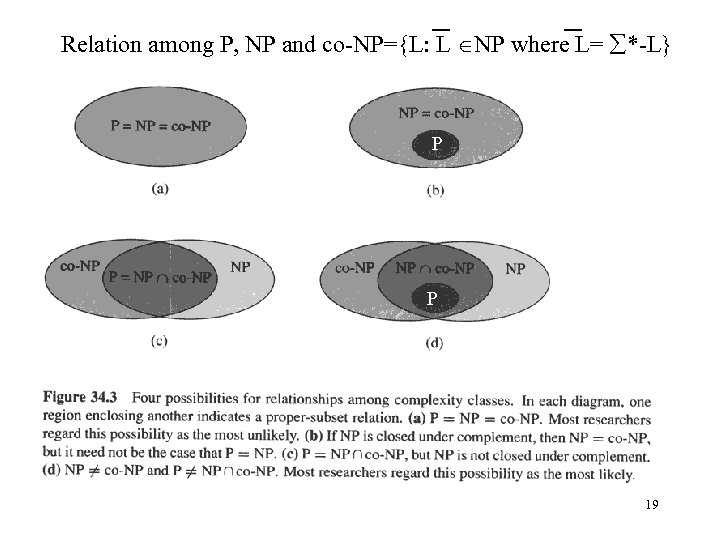Relation among P, NP and co-NP={L: L NP where L= *-L} P P 19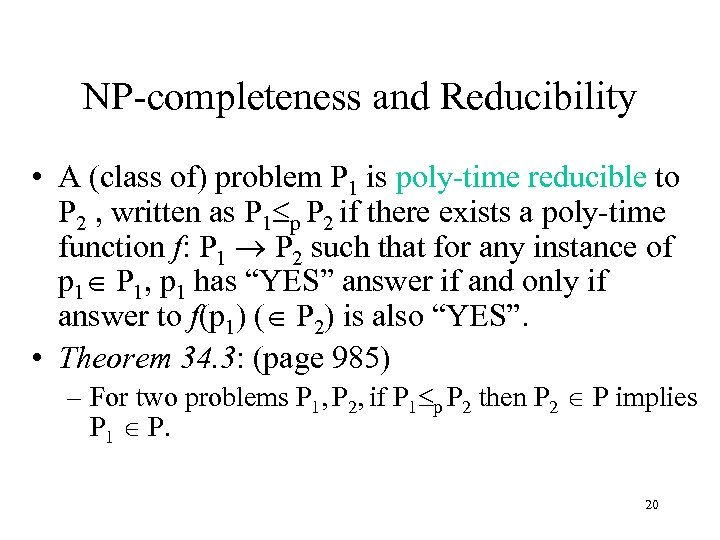NP-completeness and Reducibility • A (class of) problem P 1 is poly-time reducible to P 2 , written as P 1 p P 2 if there exists a poly-time function f: P 1 P 2 such that for any instance of p 1 P 1, p 1 has “YES” answer if and only if answer to f(p 1) ( P 2) is also “YES”. • Theorem 34. 3: (page 985) – For two problems P 1, P 2, if P 1 p P 2 then P 2 P implies P 1 P. 20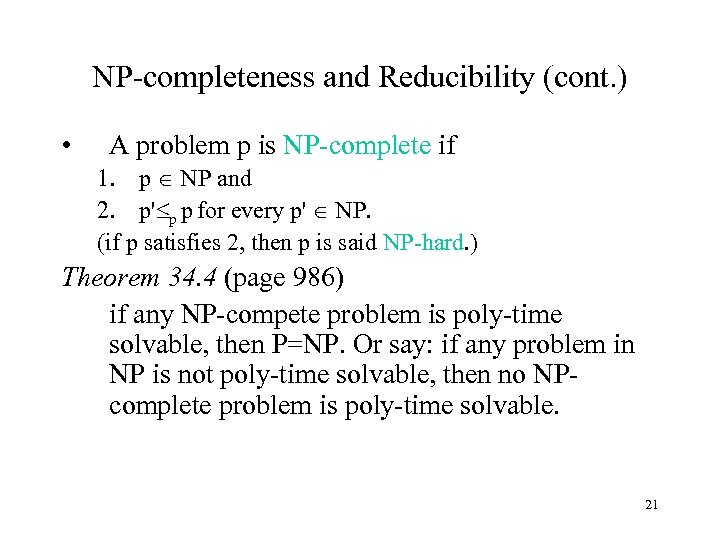NP-completeness and Reducibility (cont. ) • A problem p is NP-complete if 1. p NP and 2. p' p p for every p' NP. (if p satisfies 2, then p is said NP-hard. ) Theorem 34. 4 (page 986) if any NP-compete problem is poly-time solvable, then P=NP. Or say: if any problem in NP is not poly-time solvable, then no NPcomplete problem is poly-time solvable. 21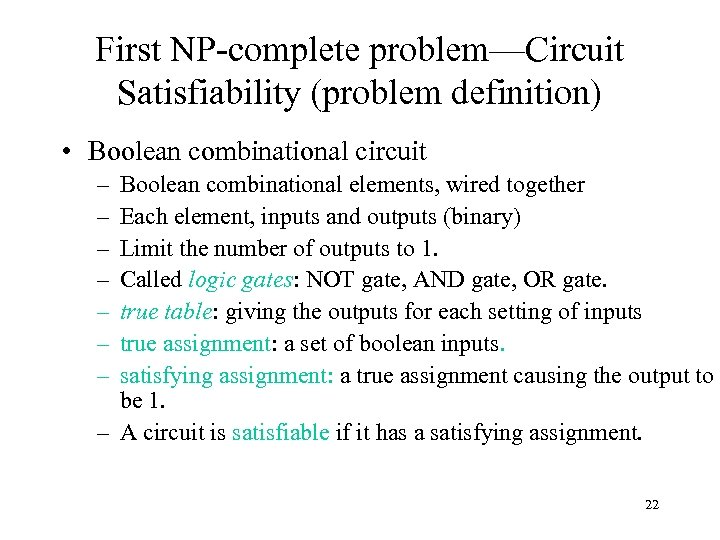First NP-complete problem—Circuit Satisfiability (problem definition) • Boolean combinational circuit – – – – Boolean combinational elements, wired together Each element, inputs and outputs (binary) Limit the number of outputs to 1. Called logic gates: NOT gate, AND gate, OR gate. true table: giving the outputs for each setting of inputs true assignment: a set of boolean inputs. satisfying assignment: a true assignment causing the output to be 1. – A circuit is satisfiable if it has a satisfying assignment. 22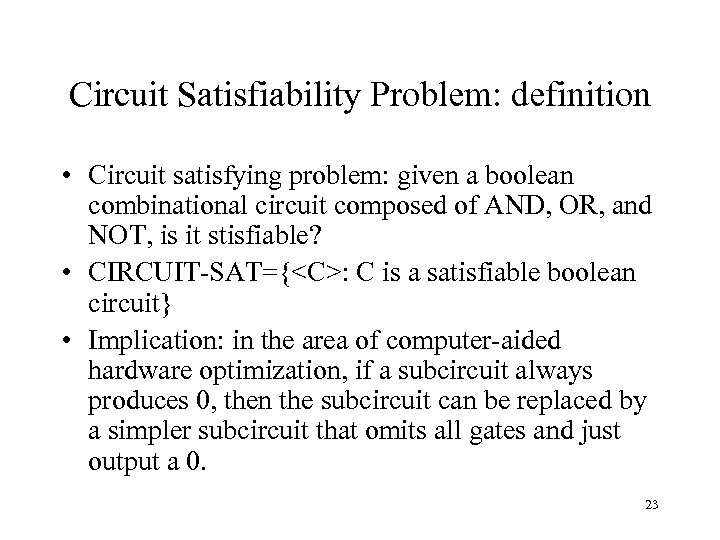Circuit Satisfiability Problem: definition • Circuit satisfying problem: given a boolean combinational circuit composed of AND, OR, and NOT, is it stisfiable? • CIRCUIT-SAT={: C is a satisfiable boolean circuit} • Implication: in the area of computer-aided hardware optimization, if a subcircuit always produces 0, then the subcircuit can be replaced by a simpler subcircuit that omits all gates and just output a 0. 23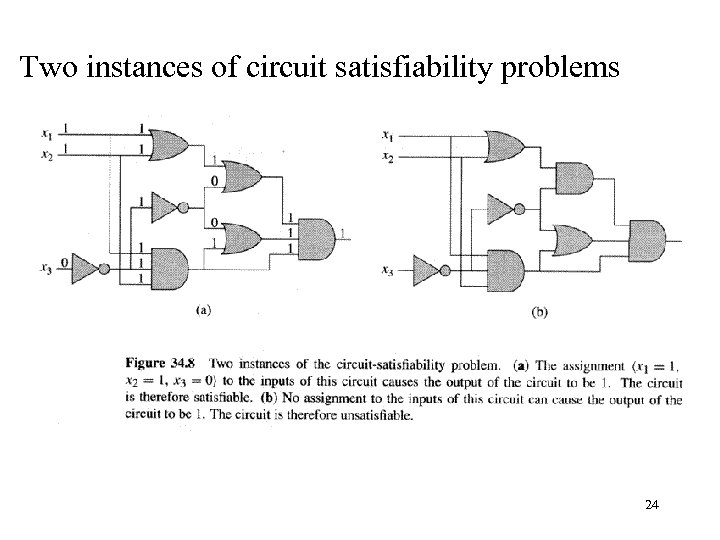Two instances of circuit satisfiability problems 24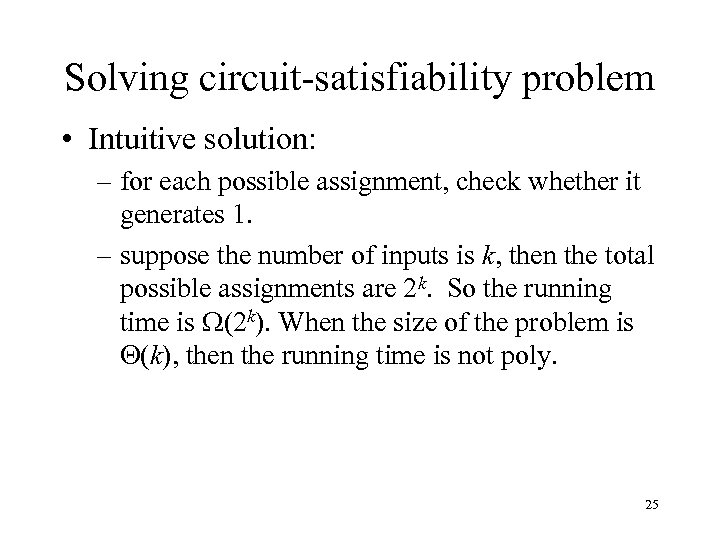Solving circuit-satisfiability problem • Intuitive solution: – for each possible assignment, check whether it generates 1. – suppose the number of inputs is k, then the total possible assignments are 2 k. So the running time is (2 k). When the size of the problem is (k), then the running time is not poly. 25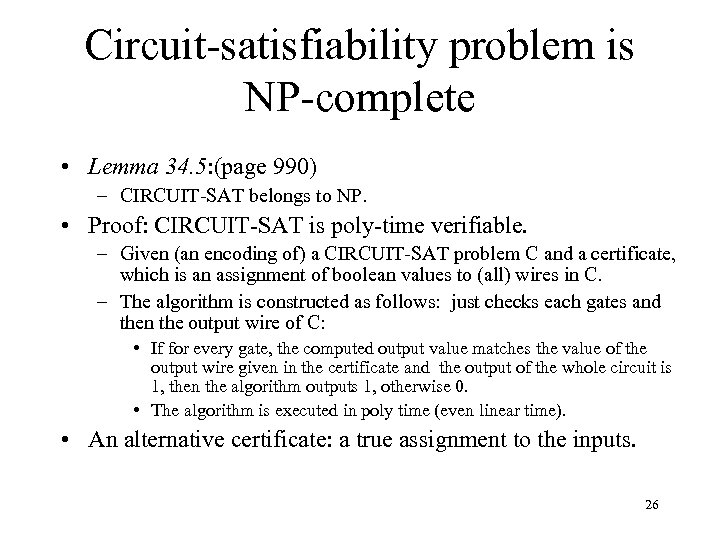Circuit-satisfiability problem is NP-complete • Lemma 34. 5: (page 990) – CIRCUIT-SAT belongs to NP. • Proof: CIRCUIT-SAT is poly-time verifiable. – Given (an encoding of) a CIRCUIT-SAT problem C and a certificate, which is an assignment of boolean values to (all) wires in C. – The algorithm is constructed as follows: just checks each gates and then the output wire of C: • If for every gate, the computed output value matches the value of the output wire given in the certificate and the output of the whole circuit is 1, then the algorithm outputs 1, otherwise 0. • The algorithm is executed in poly time (even linear time). • An alternative certificate: a true assignment to the inputs. 26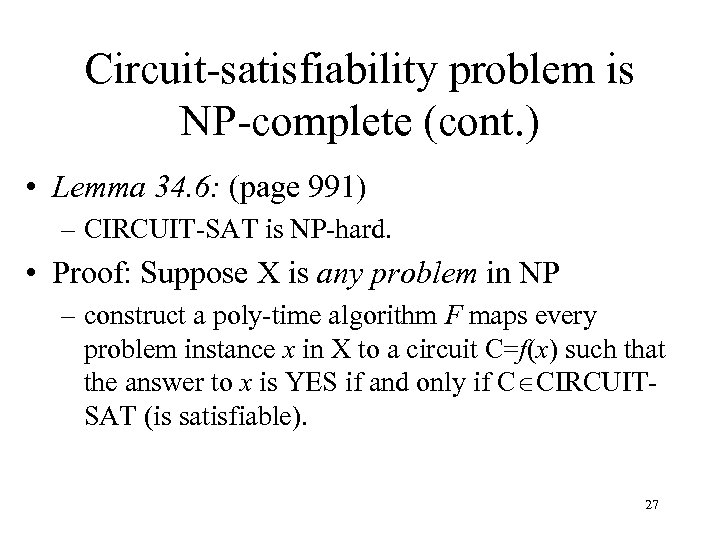Circuit-satisfiability problem is NP-complete (cont. ) • Lemma 34. 6: (page 991) – CIRCUIT-SAT is NP-hard. • Proof: Suppose X is any problem in NP – construct a poly-time algorithm F maps every problem instance x in X to a circuit C=f(x) such that the answer to x is YES if and only if C CIRCUITSAT (is satisfiable). 27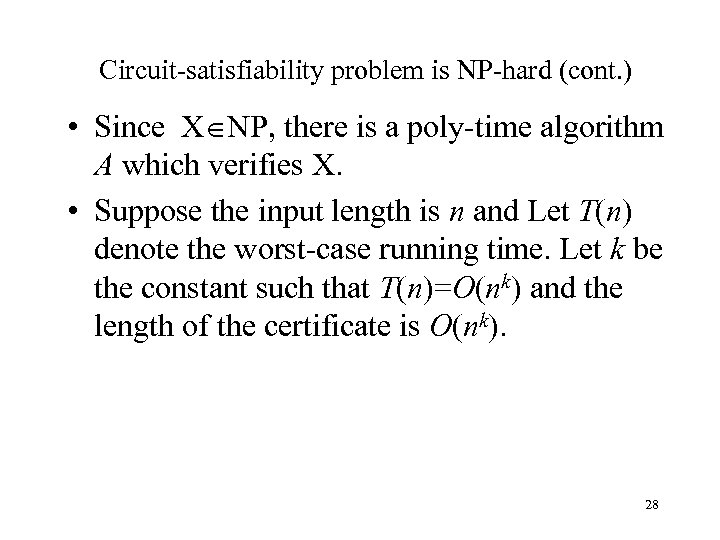Circuit-satisfiability problem is NP-hard (cont. ) • Since X NP, there is a poly-time algorithm A which verifies X. • Suppose the input length is n and Let T(n) denote the worst-case running time. Let k be the constant such that T(n)=O(nk) and the length of the certificate is O(nk). 28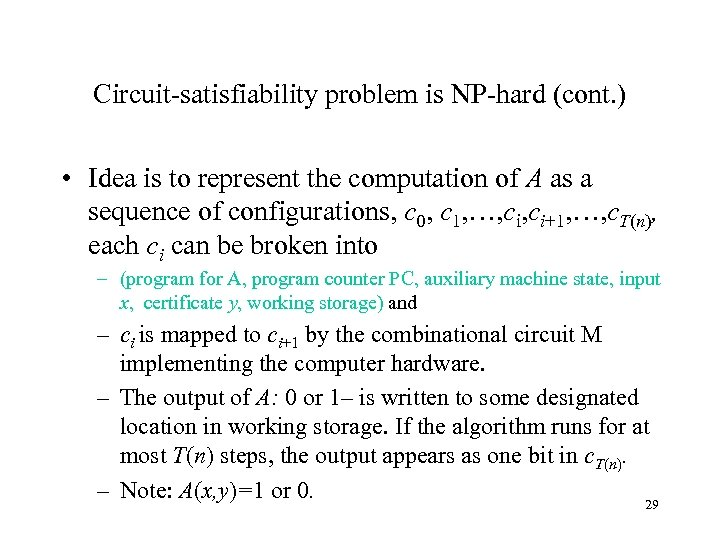Circuit-satisfiability problem is NP-hard (cont. ) • Idea is to represent the computation of A as a sequence of configurations, c 0, c 1, …, ci+1, …, c. T(n), each ci can be broken into – (program for A, program counter PC, auxiliary machine state, input x, certificate y, working storage) and – ci is mapped to ci+1 by the combinational circuit M implementing the computer hardware. – The output of A: 0 or 1– is written to some designated location in working storage. If the algorithm runs for at most T(n) steps, the output appears as one bit in c. T(n). – Note: A(x, y)=1 or 0. 29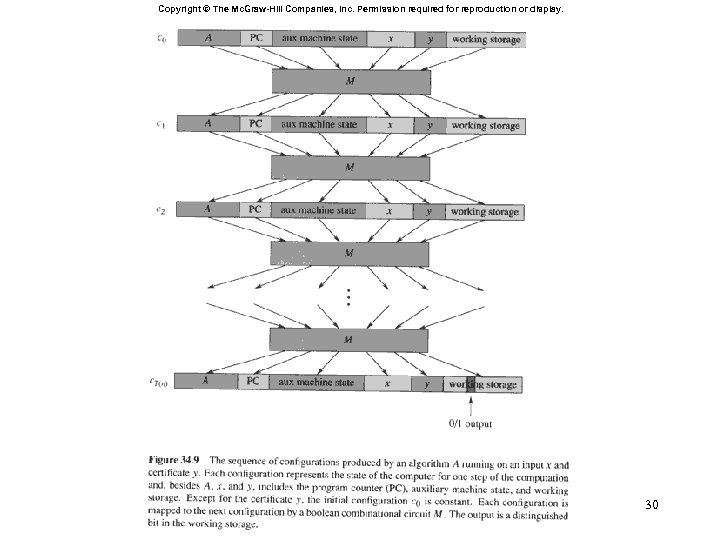Copyright © The Mc. Graw-Hill Companies, Inc. Permission required for reproduction or display. 30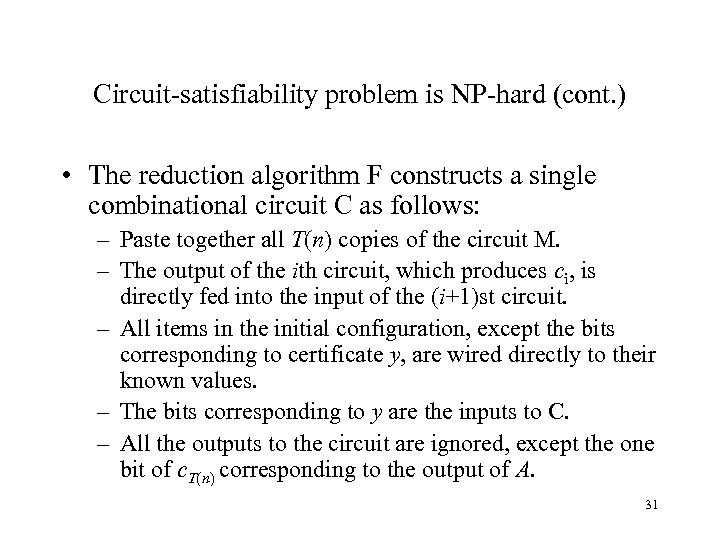Circuit-satisfiability problem is NP-hard (cont. ) • The reduction algorithm F constructs a single combinational circuit C as follows: – Paste together all T(n) copies of the circuit M. – The output of the ith circuit, which produces ci, is directly fed into the input of the (i+1)st circuit. – All items in the initial configuration, except the bits corresponding to certificate y, are wired directly to their known values. – The bits corresponding to y are the inputs to C. – All the outputs to the circuit are ignored, except the one bit of c. T(n) corresponding to the output of A. 31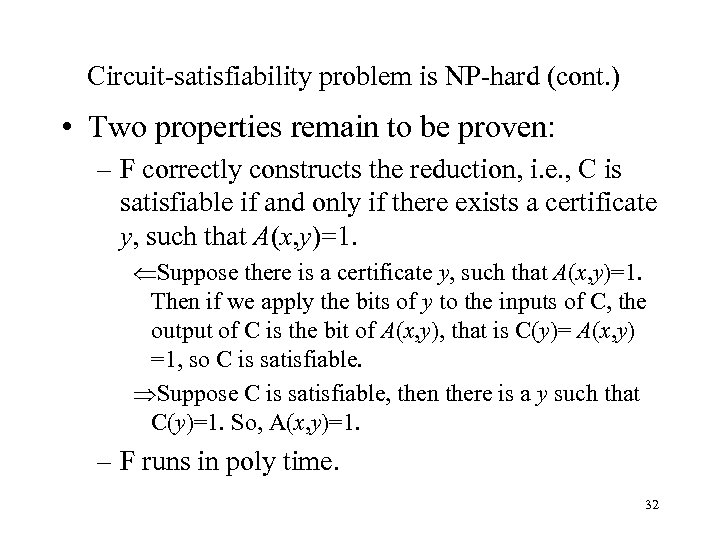Circuit-satisfiability problem is NP-hard (cont. ) • Two properties remain to be proven: – F correctly constructs the reduction, i. e. , C is satisfiable if and only if there exists a certificate y, such that A(x, y)=1. Suppose there is a certificate y, such that A(x, y)=1. Then if we apply the bits of y to the inputs of C, the output of C is the bit of A(x, y), that is C(y)= A(x, y) =1, so C is satisfiable. Suppose C is satisfiable, then there is a y such that C(y)=1. So, A(x, y)=1. – F runs in poly time. 32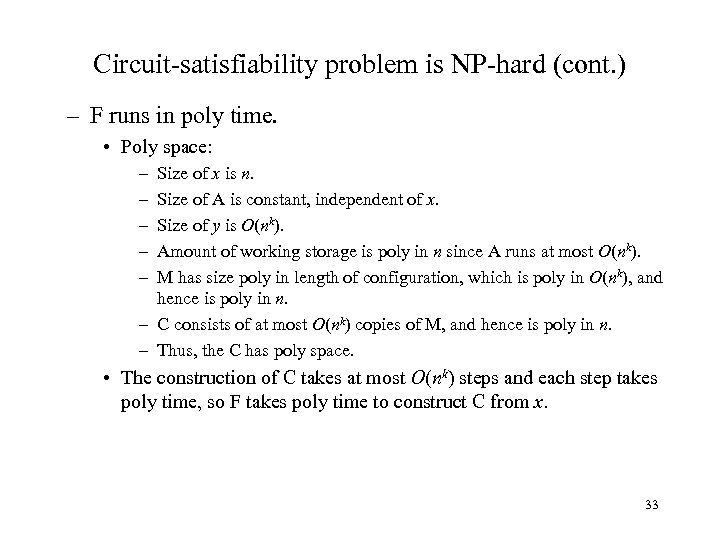Circuit-satisfiability problem is NP-hard (cont. ) – F runs in poly time. • Poly space: – – – Size of x is n. Size of A is constant, independent of x. Size of y is O(nk). Amount of working storage is poly in n since A runs at most O(nk). M has size poly in length of configuration, which is poly in O(nk), and hence is poly in n. – C consists of at most O(nk) copies of M, and hence is poly in n. – Thus, the C has poly space. • The construction of C takes at most O(nk) steps and each step takes poly time, so F takes poly time to construct C from x. 33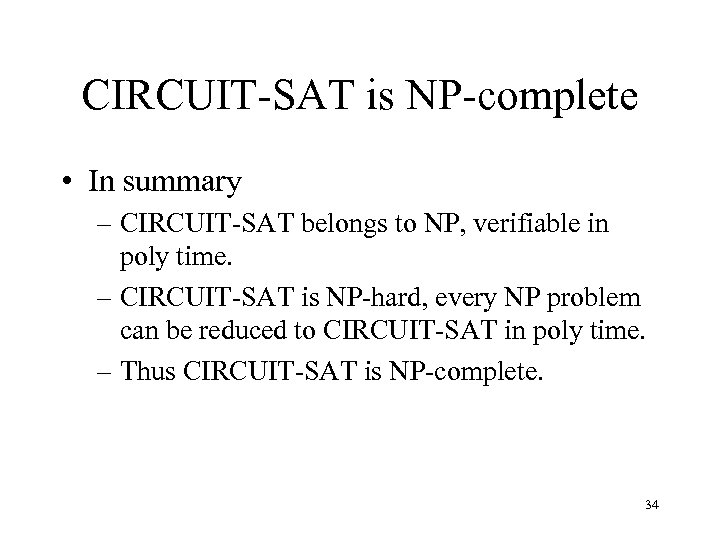CIRCUIT-SAT is NP-complete • In summary – CIRCUIT-SAT belongs to NP, verifiable in poly time. – CIRCUIT-SAT is NP-hard, every NP problem can be reduced to CIRCUIT-SAT in poly time. – Thus CIRCUIT-SAT is NP-complete. 34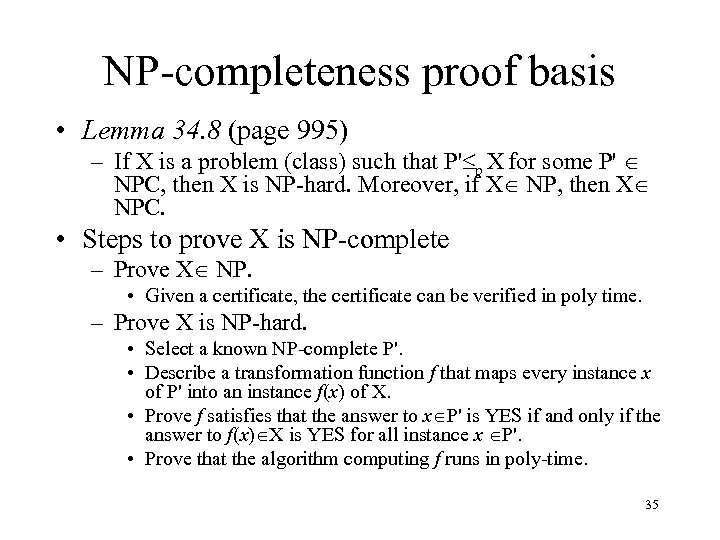NP-completeness proof basis • Lemma 34. 8 (page 995) – If X is a problem (class) such that P' p X for some P' NPC, then X is NP-hard. Moreover, if X NP, then X NPC. • Steps to prove X is NP-complete – Prove X NP. • Given a certificate, the certificate can be verified in poly time. – Prove X is NP-hard. • Select a known NP-complete P'. • Describe a transformation function f that maps every instance x of P' into an instance f(x) of X. • Prove f satisfies that the answer to x P' is YES if and only if the answer to f(x) X is YES for all instance x P'. • Prove that the algorithm computing f runs in poly-time. 35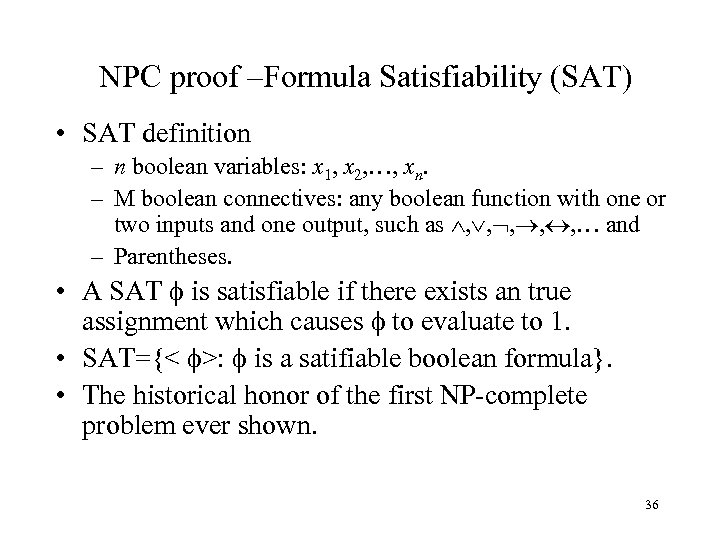NPC proof –Formula Satisfiability (SAT) • SAT definition – n boolean variables: x 1, x 2, …, xn. – M boolean connectives: any boolean function with one or two inputs and one output, such as , , , … and – Parentheses. • A SAT is satisfiable if there exists an true assignment which causes to evaluate to 1. • SAT={< >: is a satifiable boolean formula}. • The historical honor of the first NP-complete problem ever shown. 36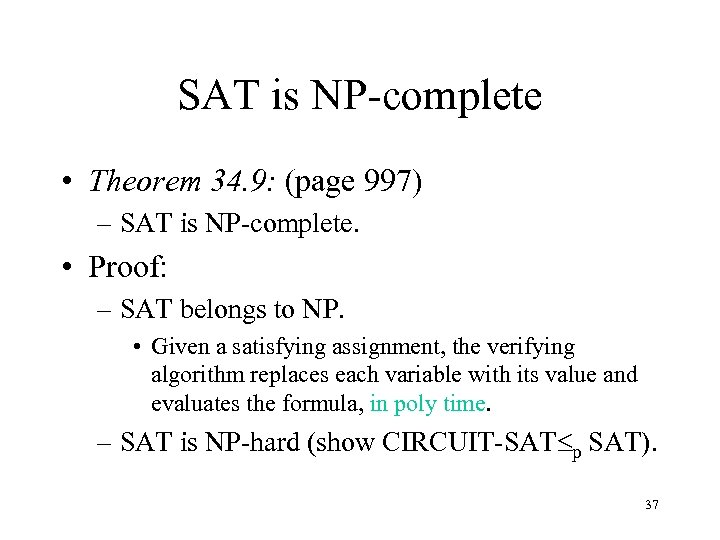SAT is NP-complete • Theorem 34. 9: (page 997) – SAT is NP-complete. • Proof: – SAT belongs to NP. • Given a satisfying assignment, the verifying algorithm replaces each variable with its value and evaluates the formula, in poly time. – SAT is NP-hard (show CIRCUIT-SAT p SAT). 37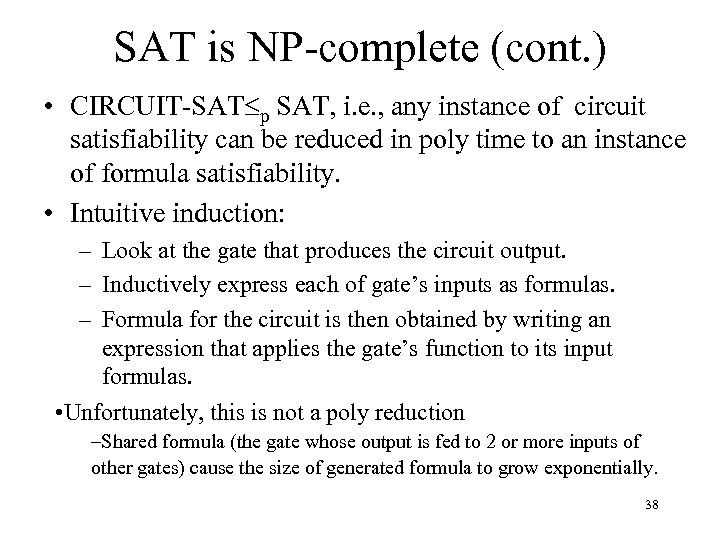SAT is NP-complete (cont. ) • CIRCUIT-SAT p SAT, i. e. , any instance of circuit satisfiability can be reduced in poly time to an instance of formula satisfiability. • Intuitive induction: – Look at the gate that produces the circuit output. – Inductively express each of gate’s inputs as formulas. – Formula for the circuit is then obtained by writing an expression that applies the gate’s function to its input formulas. • Unfortunately, this is not a poly reduction –Shared formula (the gate whose output is fed to 2 or more inputs of other gates) cause the size of generated formula to grow exponentially. 38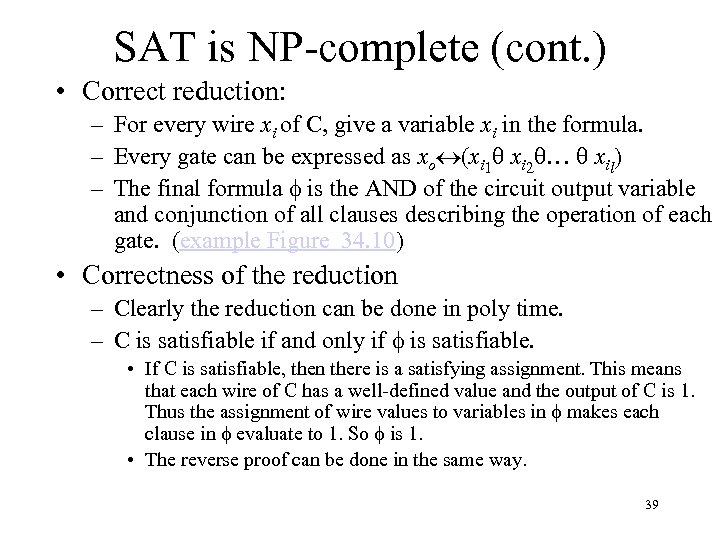SAT is NP-complete (cont. ) • Correct reduction: – For every wire xi of C, give a variable xi in the formula. – Every gate can be expressed as xo (xi 1 xi 2 … xil) – The final formula is the AND of the circuit output variable and conjunction of all clauses describing the operation of each gate. (example Figure 34. 10) • Correctness of the reduction – Clearly the reduction can be done in poly time. – C is satisfiable if and only if is satisfiable. • If C is satisfiable, then there is a satisfying assignment. This means that each wire of C has a well-defined value and the output of C is 1. Thus the assignment of wire values to variables in makes each clause in evaluate to 1. So is 1. • The reverse proof can be done in the same way. 39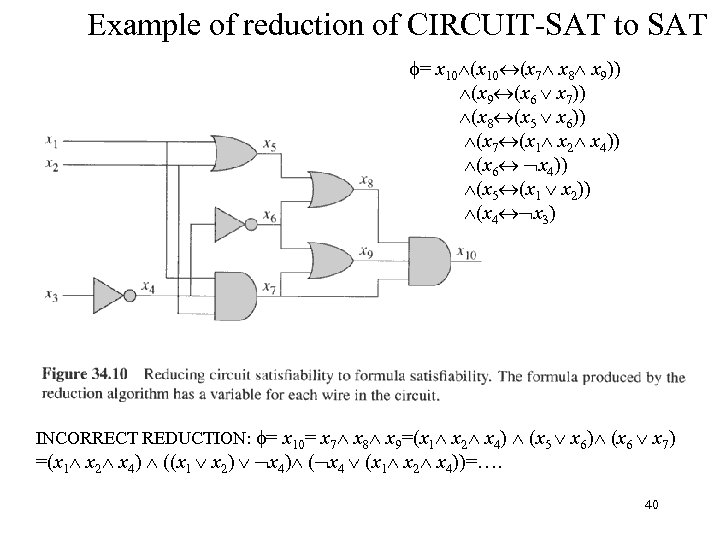Example of reduction of CIRCUIT-SAT to SAT = x 10 (x 7 x 8 x 9)) (x 9 (x 6 x 7)) (x 8 (x 5 x 6)) (x 7 (x 1 x 2 x 4)) (x 6 x 4)) (x 5 (x 1 x 2)) (x 4 x 3) INCORRECT REDUCTION: = x 10= x 7 x 8 x 9=(x 1 x 2 x 4) (x 5 x 6) (x 6 x 7) =(x 1 x 2 x 4) ((x 1 x 2) x 4) ( x 4 (x 1 x 2 x 4))=…. 40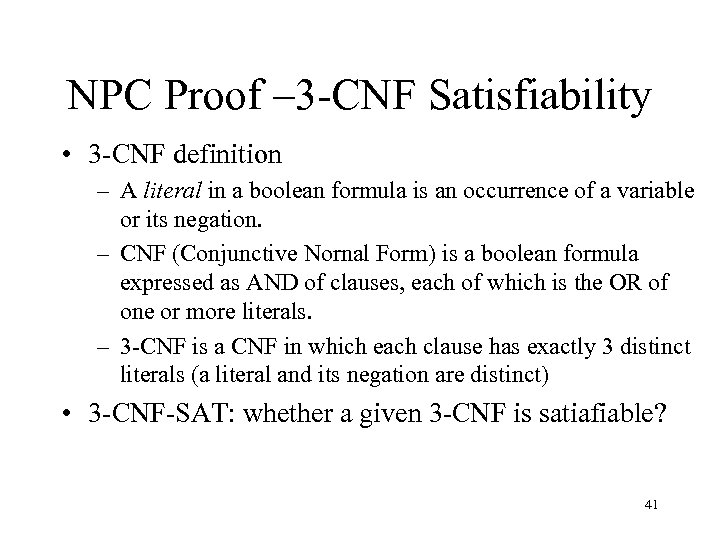NPC Proof – 3 -CNF Satisfiability • 3 -CNF definition – A literal in a boolean formula is an occurrence of a variable or its negation. – CNF (Conjunctive Nornal Form) is a boolean formula expressed as AND of clauses, each of which is the OR of one or more literals. – 3 -CNF is a CNF in which each clause has exactly 3 distinct literals (a literal and its negation are distinct) • 3 -CNF-SAT: whether a given 3 -CNF is satiafiable? 41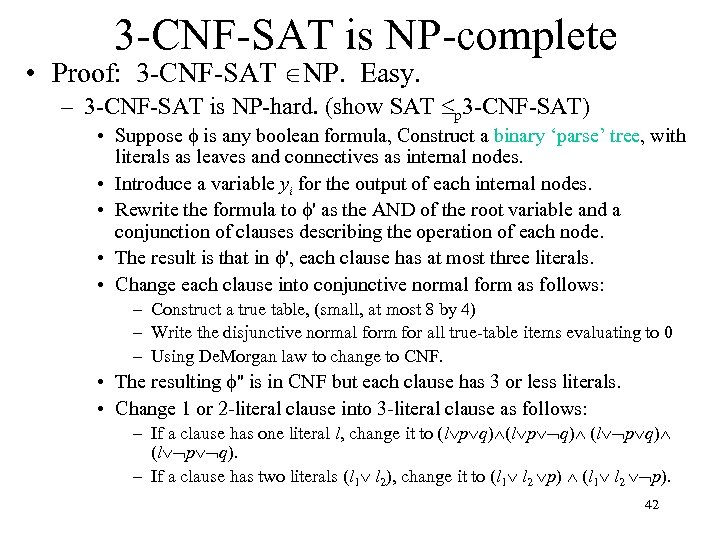3 -CNF-SAT is NP-complete • Proof: 3 -CNF-SAT NP. Easy. – 3 -CNF-SAT is NP-hard. (show SAT p 3 -CNF-SAT) • Suppose is any boolean formula, Construct a binary ‘parse’ tree, with literals as leaves and connectives as internal nodes. • Introduce a variable yi for the output of each internal nodes. • Rewrite the formula to ' as the AND of the root variable and a conjunction of clauses describing the operation of each node. • The result is that in ', each clause has at most three literals. • Change each clause into conjunctive normal form as follows: – Construct a true table, (small, at most 8 by 4) – Write the disjunctive normal form for all true-table items evaluating to 0 – Using De. Morgan law to change to CNF. • The resulting '' is in CNF but each clause has 3 or less literals. • Change 1 or 2 -literal clause into 3 -literal clause as follows: – If a clause has one literal l, change it to (l p q). – If a clause has two literals (l 1 l 2), change it to (l 1 l 2 p) (l 1 l 2 p). 42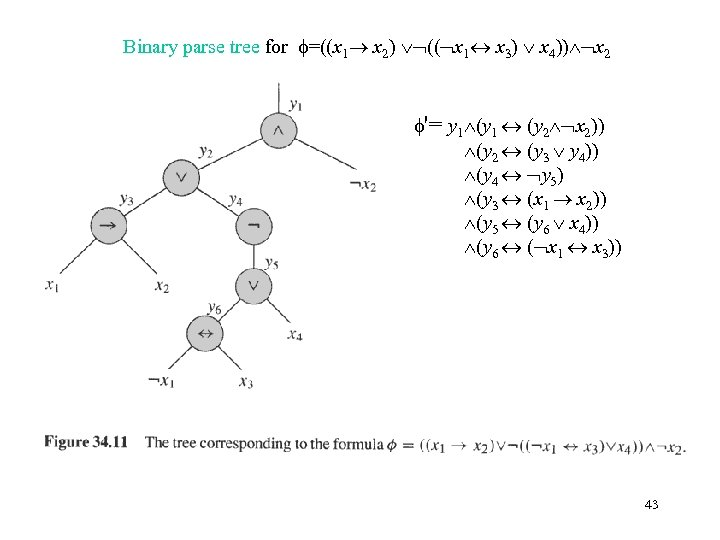Binary parse tree for =((x 1 x 2) (( x 1 x 3) x 4)) x 2 '= y 1 (y 1 (y 2 x 2)) (y 2 (y 3 y 4)) (y 4 y 5) (y 3 (x 1 x 2)) (y 5 (y 6 x 4)) (y 6 ( x 1 x 3)) 43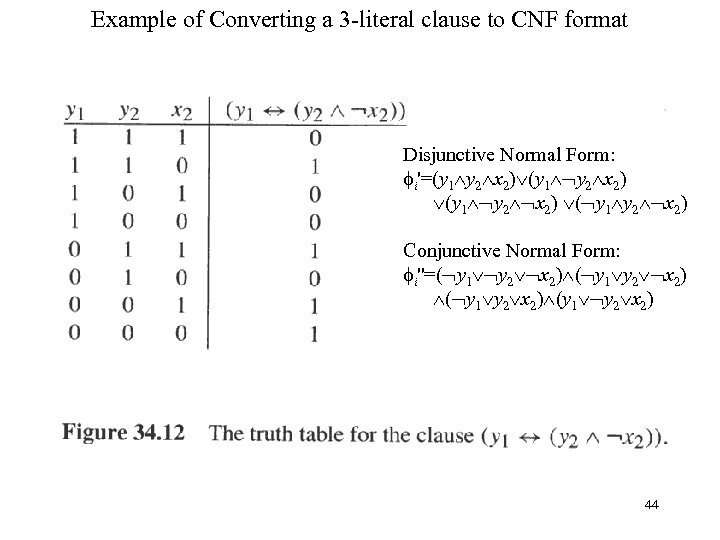Example of Converting a 3 -literal clause to CNF format Disjunctive Normal Form: i'=(y 1 y 2 x 2) ( y 1 y 2 x 2) Conjunctive Normal Form: i''=( y 1 y 2 x 2) (y 1 y 2 x 2) 44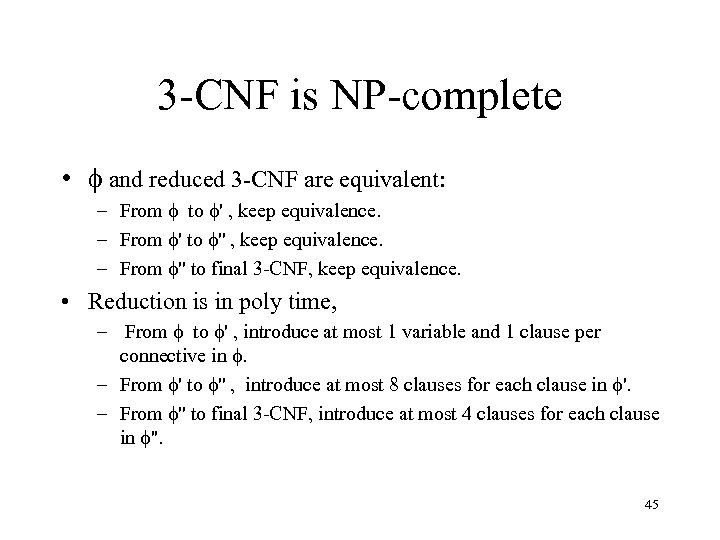3 -CNF is NP-complete • and reduced 3 -CNF are equivalent: – From to ' , keep equivalence. – From ' to '' , keep equivalence. – From '' to final 3 -CNF, keep equivalence. • Reduction is in poly time, – From to ' , introduce at most 1 variable and 1 clause per connective in . – From ' to '' , introduce at most 8 clauses for each clause in '. – From '' to final 3 -CNF, introduce at most 4 clauses for each clause in ''. 45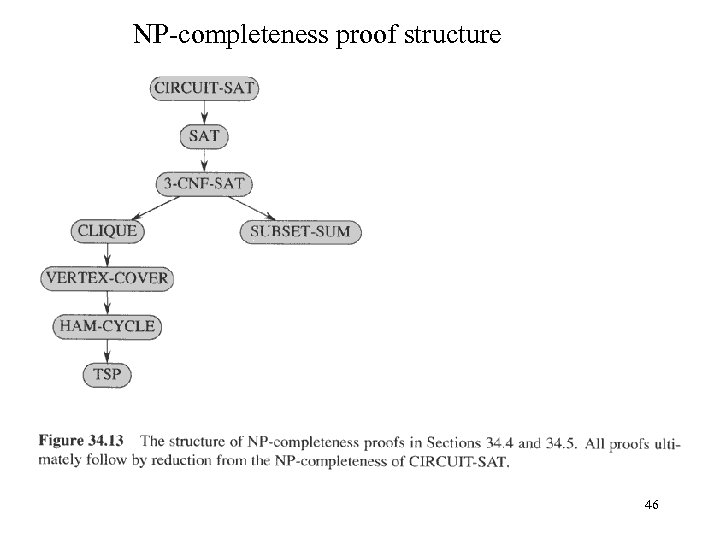NP-completeness proof structure 46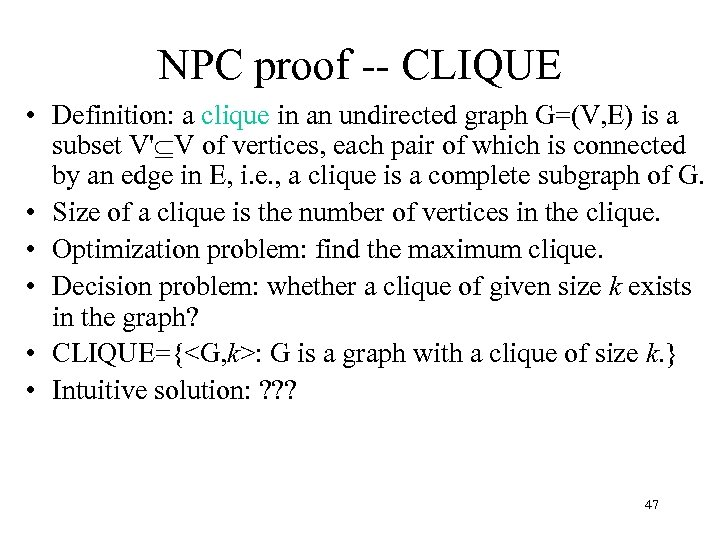NPC proof -- CLIQUE • Definition: a clique in an undirected graph G=(V, E) is a subset V' V of vertices, each pair of which is connected by an edge in E, i. e. , a clique is a complete subgraph of G. • Size of a clique is the number of vertices in the clique. • Optimization problem: find the maximum clique. • Decision problem: whether a clique of given size k exists in the graph? • CLIQUE={: G is a graph with a clique of size k. } • Intuitive solution: ? ? ? 47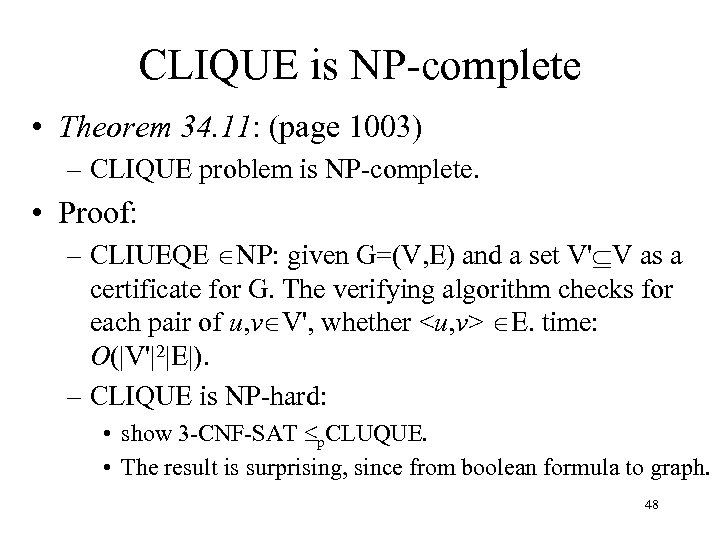CLIQUE is NP-complete • Theorem 34. 11: (page 1003) – CLIQUE problem is NP-complete. • Proof: – CLIUEQE NP: given G=(V, E) and a set V' V as a certificate for G. The verifying algorithm checks for each pair of u, v V', whether E. time: O(|V'|2|E|). – CLIQUE is NP-hard: • show 3 -CNF-SAT p. CLUQUE. • The result is surprising, since from boolean formula to graph. 48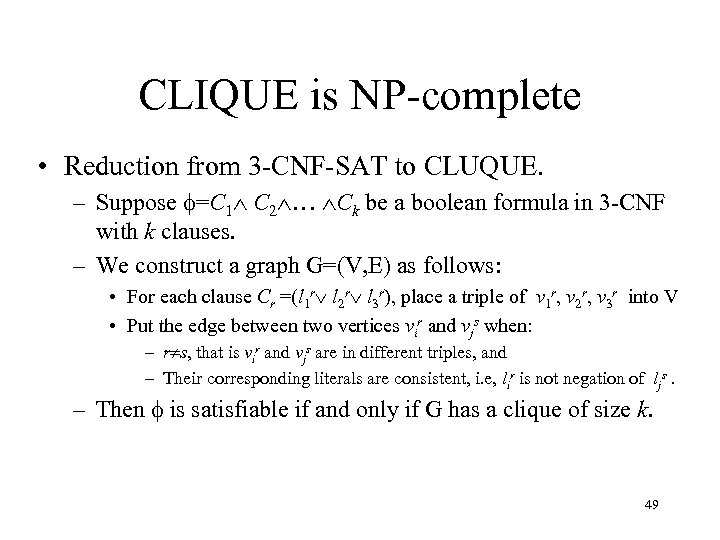CLIQUE is NP-complete • Reduction from 3 -CNF-SAT to CLUQUE. – Suppose =C 1 C 2 … Ck be a boolean formula in 3 -CNF with k clauses. – We construct a graph G=(V, E) as follows: • For each clause Cr =(l 1 r l 2 r l 3 r), place a triple of v 1 r, v 2 r, v 3 r into V • Put the edge between two vertices vir and vjs when: – r s, that is vir and vjs are in different triples, and – Their corresponding literals are consistent, i. e, lir is not negation of ljs. – Then is satisfiable if and only if G has a clique of size k. 49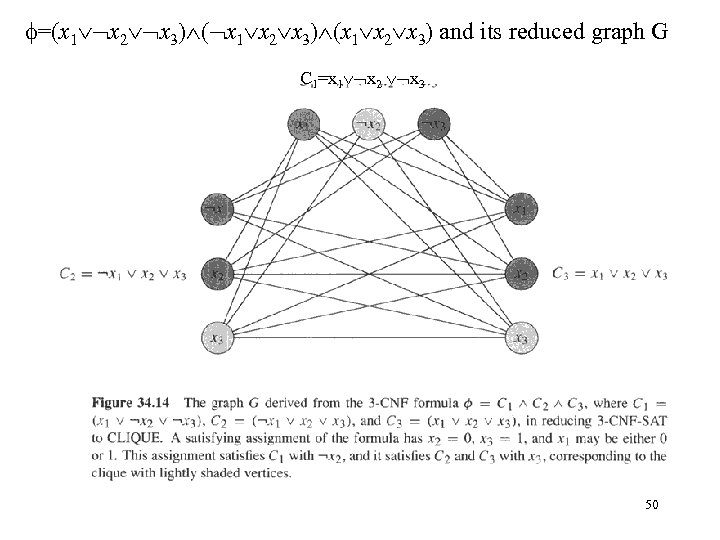=(x 1 x 2 x 3) (x 1 x 2 x 3) and its reduced graph G C 1=x 1 x 2 x 3 50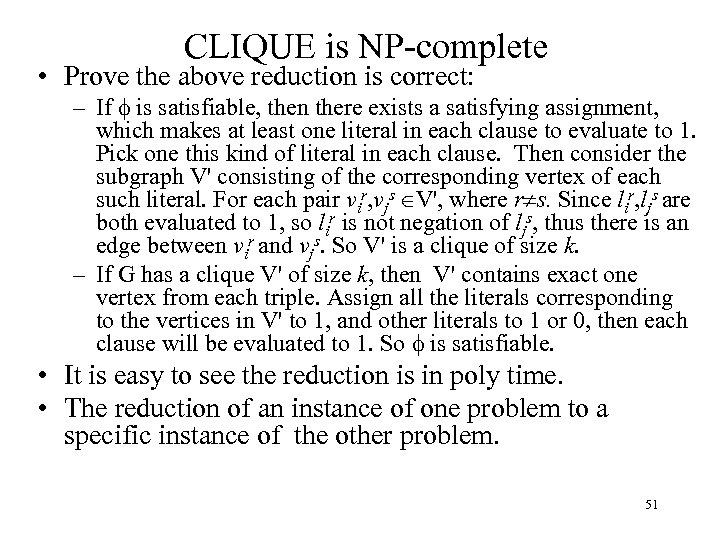CLIQUE is NP-complete • Prove the above reduction is correct: – If is satisfiable, then there exists a satisfying assignment, which makes at least one literal in each clause to evaluate to 1. Pick one this kind of literal in each clause. Then consider the subgraph V' consisting of the corresponding vertex of each such literal. For each pair vir, vjs V', where r s. Since lir, ljs are both evaluated to 1, so lir is not negation of ljs, thus there is an edge between vir and vjs. So V' is a clique of size k. – If G has a clique V' of size k, then V' contains exact one vertex from each triple. Assign all the literals corresponding to the vertices in V' to 1, and other literals to 1 or 0, then each clause will be evaluated to 1. So is satisfiable. • It is easy to see the reduction is in poly time. • The reduction of an instance of one problem to a specific instance of the other problem. 51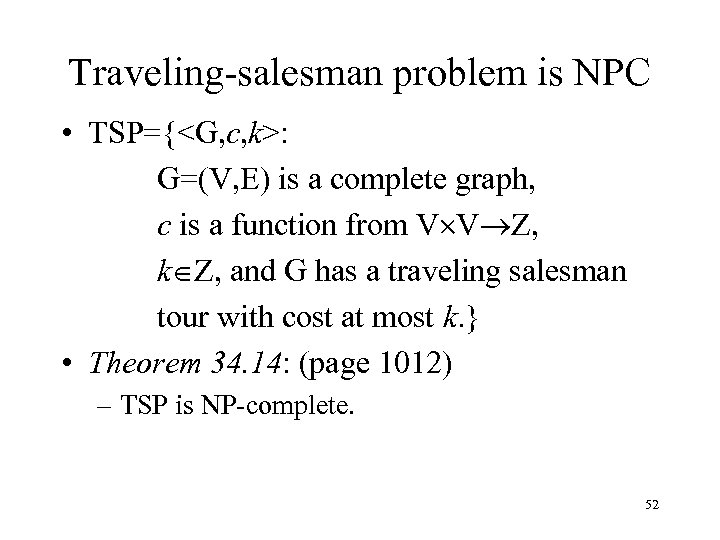Traveling-salesman problem is NPC • TSP={: G=(V, E) is a complete graph, c is a function from V V Z, k Z, and G has a traveling salesman tour with cost at most k. } • Theorem 34. 14: (page 1012) – TSP is NP-complete. 52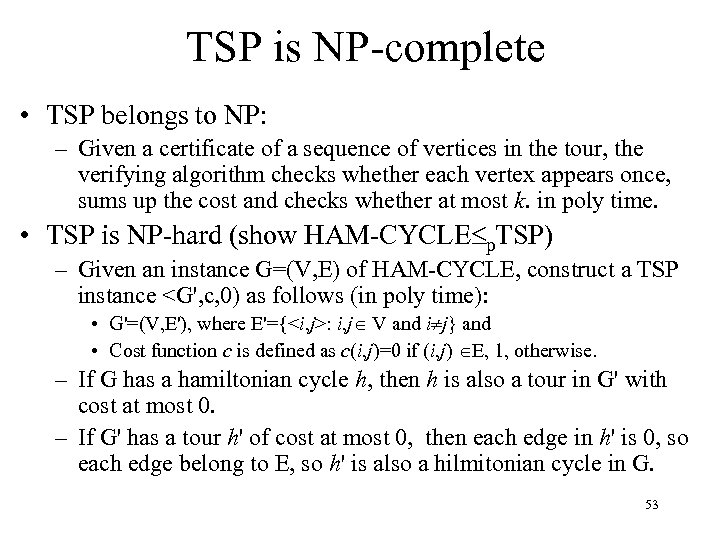TSP is NP-complete • TSP belongs to NP: – Given a certificate of a sequence of vertices in the tour, the verifying algorithm checks whether each vertex appears once, sums up the cost and checks whether at most k. in poly time. • TSP is NP-hard (show HAM-CYCLE p. TSP) – Given an instance G=(V, E) of HAM-CYCLE, construct a TSP instance : i, j V and i j} and • Cost function c is defined as c(i, j)=0 if (i, j) E, 1, otherwise. – If G has a hamiltonian cycle h, then h is also a tour in G' with cost at most 0. – If G' has a tour h' of cost at most 0, then each edge in h' is 0, so each edge belong to E, so h' is also a hilmitonian cycle in G. 53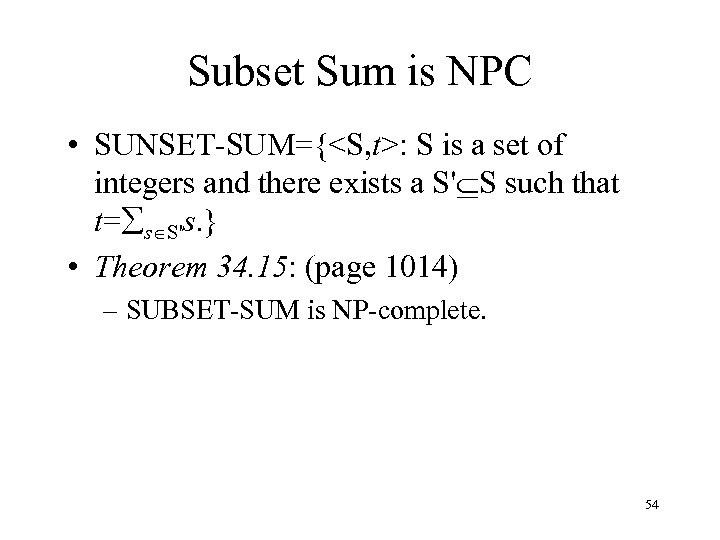Subset Sum is NPC • SUNSET-SUM={: S is a set of integers and there exists a S' S such that t= s S's. } • Theorem 34. 15: (page 1014) – SUBSET-SUM is NP-complete. 54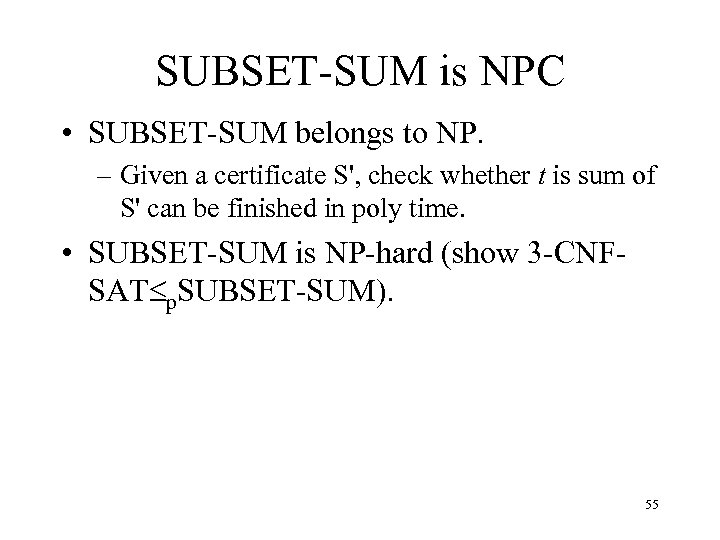SUBSET-SUM is NPC • SUBSET-SUM belongs to NP. – Given a certificate S', check whether t is sum of S' can be finished in poly time. • SUBSET-SUM is NP-hard (show 3 -CNFSAT p. SUBSET-SUM). 55SUBSET-SUM is NPC • Given a 3 -CNF formula =C 1 C 2 … Ck with literals x 1, x 2, …, xn. Construct a SUBSET-SUM instance as follows: – Two assumptions: no clause contains both a literal and its negation, and either a literal or its negation appears in at least one clause. – The numbers in S are based on 10 and have n+k digits, each digit corresponds to (or is labeled by) a literal or a clause. – Target t=1… 1||4… 4 (n 1’s and k 4’s) – For each literal xi, create two integers: • vi=0… 01(i)0… 0||0… 01(l)0… 01(w)0… 0, where xi appears in Cl, …, Cw. • vi'=0… 01(i)0… 0||0… 1(m)0…… 01(p)0… 0, where xi appears in Cm, …, Cp. . – • Clearly, vi and vi' can not be equal in right k digits, moreover all vi and vi' in S are distinct. For each clause Cj, create two integers: • sj=0… 0||0… 01(j)0… 0, • sj'=0… 0||0… 02(j)0… 0. • all sj and sj' are called “slack number”. Clearly, all sj and sj' in S are distinct. – Note: the sum of digits in any one digit position is 2 or 6, so when there is no carries when adding any subset of the above integers. 56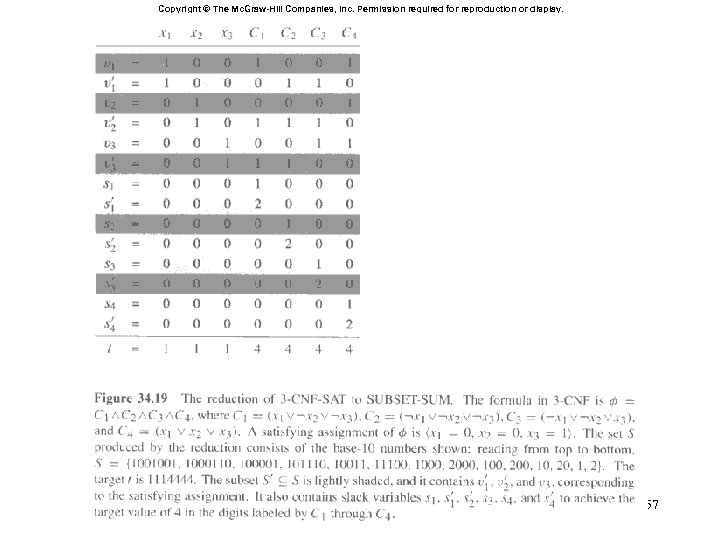Copyright © The Mc. Graw-Hill Companies, Inc. Permission required for reproduction or display. 57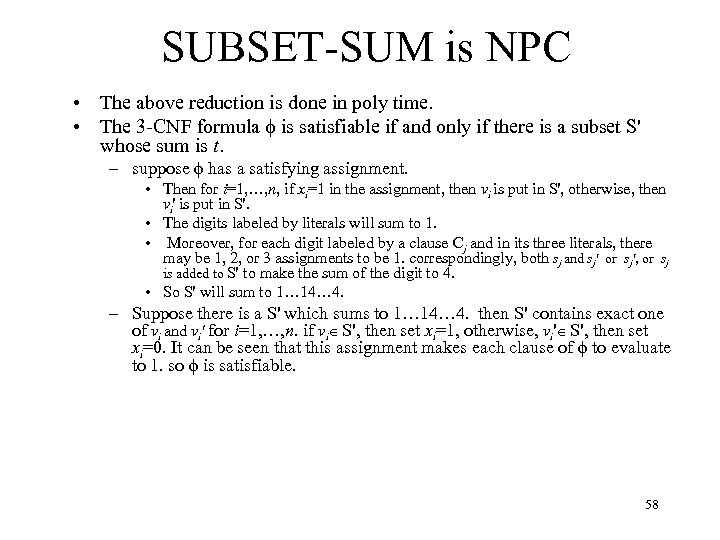SUBSET-SUM is NPC • The above reduction is done in poly time. • The 3 -CNF formula is satisfiable if and only if there is a subset S' whose sum is t. – suppose has a satisfying assignment. • Then for i=1, …, n, if xi=1 in the assignment, then vi is put in S', otherwise, then vi' is put in S'. • The digits labeled by literals will sum to 1. • Moreover, for each digit labeled by a clause Cj and in its three literals, there may be 1, 2, or 3 assignments to be 1. correspondingly, both sj and sj' or sj', or sj is added to S' to make the sum of the digit to 4. • So S' will sum to 1… 14… 4. – Suppose there is a S' which sums to 1… 14… 4. then S' contains exact one of vi and vi' for i=1, …, n. if vi S', then set xi=1, otherwise, vi' S', then set xi=0. It can be seen that this assignment makes each clause of to evaluate to 1. so is satisfiable. 58